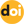Izvestiya vuzov. Yadernaya Energetika

The peer-reviewed scientific and technology journal. ISSN: 0204-3327

# Solution of the two-dimensional problem of non-stationary thermal conductivity in a k-layer plate and cylinderhttps://doi.org/10.26583/npe.2020.1.06

### UDC: 536.21

The problem of determining a two-dimensional non-stationary temperature field in a k-layer cylinder and plate of length l is solved. There is a symmetrically located gap (plate) or cylindrical cavity (cylinder) in the center of these bodies. The absence of a gap or cavity is a special case of the problem. In each layer, there are heat sources, depending on the coordinates and time.

The initial temperature of the layers is a function of the coordinates. In the center of the bodies the symmetry condition is fulfilled. At the boundary of contact of the layers – ideal thermal contact: continuity of temperatures and heat flows. On the outer side surface and ends, heat exchange occurs according to Newton’s law with environments whose temperatures change over time according to an arbitrary law. With the help of the geometric parameter Γ in the mathematical formulation of the problem, one differential equation for both multilayer bodies is written. The problem in this statement is solved for the first time.

To solve the problem, a combined method is used: first, by using the method of finite integral transformations, differential operations on the longitudinal coordinate are excluded, and then the resulting equation in the images is solved by the Fourier method (separation of variables), bringing the determination of the time dependence of the temperature to the solution of an ordinary differential equation of first order.

1. Lykov A.V. Theory of Thermal Conductivity. Moscow. Vysshaya Shkola Publ., 1967. 600 p. Avaiable at: http://bookre.org/reader?file=454814 (accessed Dec 09, 2019) (in Russian).
2. Kartashov E.M. Analytical Methods in the Theory of Thermal Conductivity of Solids. Textbook. Moscow. Vysshaya Shkola Publ., 2001. 550 p. (in Russian).
3. Butkovskij A.G. Characteristics of Systems with Distributed Parameters. Moscow. Nauka Publ., 1979. 224 p. (in Russian).
4. Budak B.M., Samarskij A.A., Tihonov A.N. Collection of Problems in Mathematical Physics. Moscow. Nauka Publ., 1980. 688 p. (in Russian).
5. Smirnov M.S. The Problem of Thermal Conductivity for a System of Two Bodies. In the book «Heat and mass transfer in evaporation processes». Moscow. Akademiya nauk SSSR Publ., 1958, pp. 153-155 (in Russian).
6. Smirnov M.S. Temperature Field in a Three+Layer Wall under Boundary Conditions of the Fourth Kind. In the book «Heat and mass transfer in capillary-porous bodies». Moscow-Leningrad. Gosenergoizdat Publ., 1957, pp. 17-20 (in Russian).
7. Bulavin P.E., Kascheev V.M. The solution of the inhomogeneous heat conduction equation for multilayered bodies. Inzhenerno+Fizicheskij Zhurnal. 1964, v. VII, no. 9, pp. 71-77 (in Russian).
8. Tugolukov E. N. Solution of Heat Conduction Problems by the Method of Finite Integral Transformations. Textbook. Tambov. TGTU Publ., 2005. 116 p. (in Russian).
9. Gulevich A.V., D’yachenko P.P., Zrodnikov A.V., Kuharchuk O.F. Coupled Reactor Systems of Pulse Action. Moscow. Energoatomizdat Publ., 2003. 360 p. (in Russian).
10. Koshlyakov N.S., Gliner E.B., Smirnov M.M. Differential Equations of Mathematical Physics. Moscow. FIZMATGIZ Publ., 1962. 730 p. (in Russian).
11. Tikhonov A.N., Samarsky A.A. Equations of Mathematical Physics. Ed. 7, stereo. Moscow. MGU Publ., 2004, 800 p. ISBN 5-211-04843-1 (in Russian).
12. Kurosh A.G. Higher Algebra Course. Ed. 15, stereo. Saint-Petersburg. Lan’ Publ., 2006. 432 p. ISBN 978-5-8114-0521-3. (in Russian).